Updated date:

# Fascinating world of Leonardo Fibonacci numbers

Author:

What is the main reason of Mona Lisa’s attractiveness and an exceptional beauty? Whether the secret is in her smile or simple in Leonardo da Vinci’s excellence in painting? One can assume more possible answers and comments on these questions, but if I ask what Mona Lisa and Parthenon have in common, than the space for answers is narrowed... and it can be guest that I mean on special proportion among the act parts, known as the golden ratio (also called the divine proportion).

However in this article we don’t go into questions like can beauty be hidden in numbers, rather I will give an introduction into subject of Fibonacci numbers as well as to point out its connection to the golden ratio. Although this overview of Fibonacci numbers is concise it is quite informative serving as a good starting point to magnificent world of Fibonacci numbers and its relation with nature, science and art.

## Leonardo Pisano Fibonacci

Leonardo Fibonacci is considered as the greatest European mathematicians of the Middle Ages. He is largely responsible for introducing the Arabic numerals, about which he writes in his most important work “Liber abaci” (the book of the abacus) from the 1202nd year. Nevertheless, his name is usually associated with a series of natural numbers

1, 1, 2, 3, 5, 8, 13, 21, 34, 55, 89,…

whose members are called Fibonacci numbers.

Fibonacci was born in Pisa, a city with well-known inclined tower. His father (Guglielmo Bonaccio) performed his diplomatic service in the North African city Bugia, and he took Leonardo with him. As Fibonacci with his father traveled throughout the Mediterranean ports, he met the Arab way of recording numbers using 10 digits and very soon he noticed its advantage. Thus Fibonacci wrote about it in the Liber abaci:

When my father, who had been appointed by his country as public notary in the customs at Bugia acting for the Pisan merchants going there, was in charge, he summoned me to him while I was still a child, and having an eye to usefulness and future convenience, desired me to stay there and receive instruction in the school of accounting. There, when I had been introduced to the art of the Indian’s nine symbols through remarkable teaching, knowledge of art in Egypt, Syria, Greece, Sicily and Provence, in all its various forms.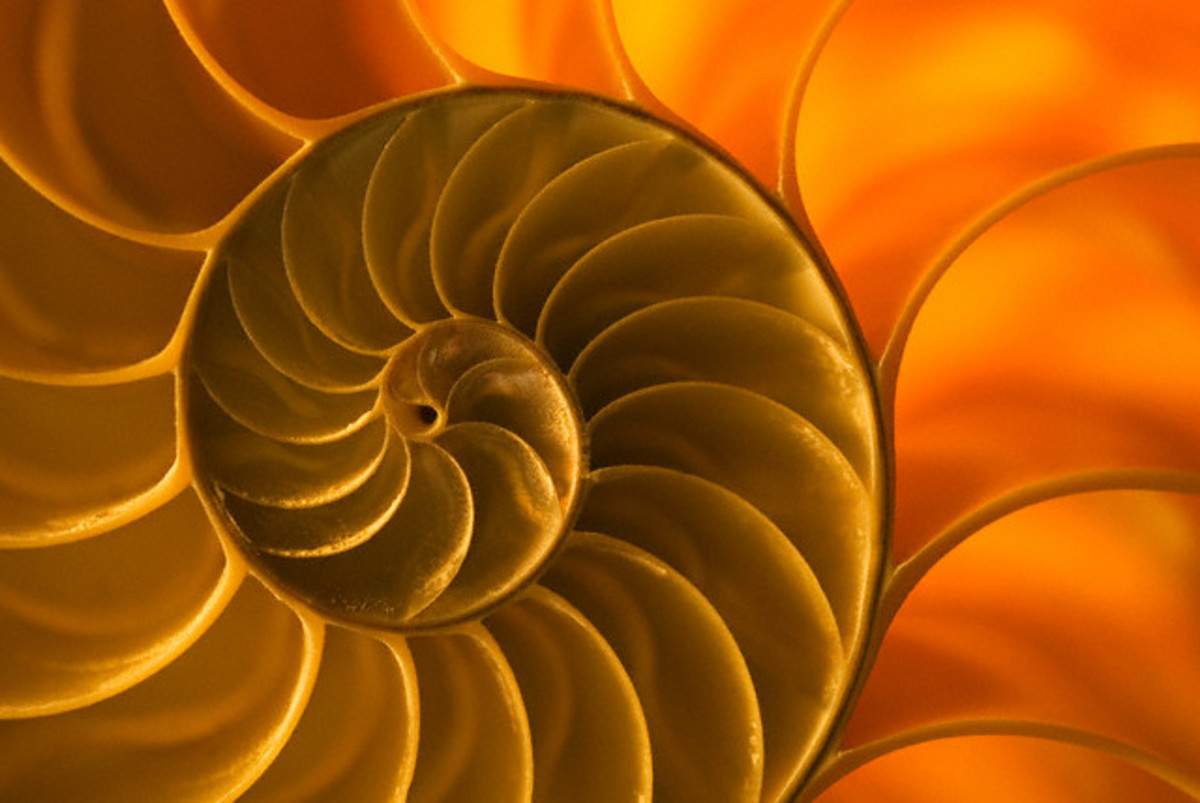Fig. 1. Nautilus shall. The pattern of Fibonacci spiral is ubiquitous in the nature, including nautilus stall and galaxies.

Around 100 AD Fibonacci returned to Pisa and began with scientific work. Since he lived in the time before the invention of the printing machine, he wrote his books manually. His the most important works are: Liber abaci (1202.), Practica geometriae (1220.), Flos (1225.), Liber quadratorum.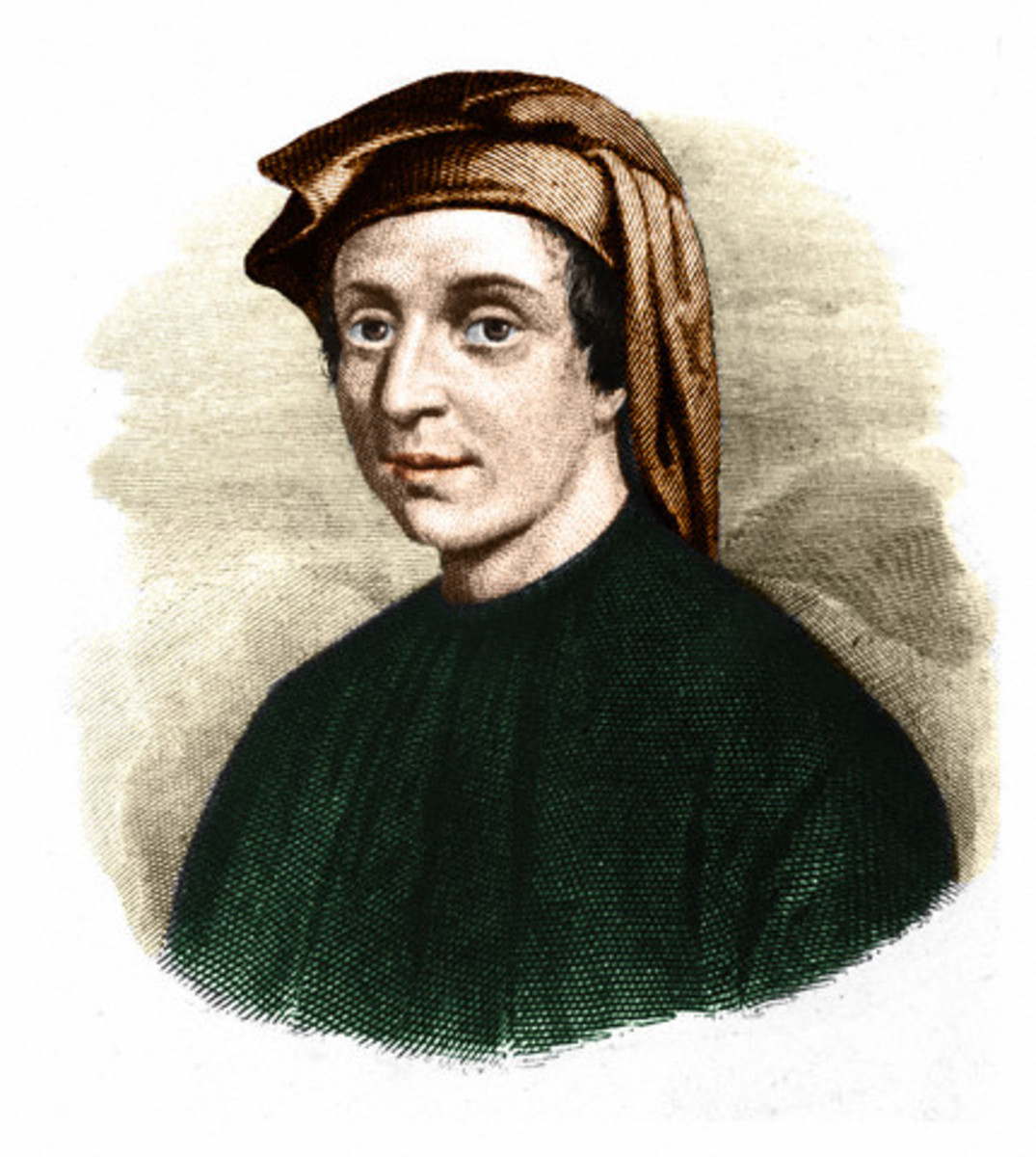Fig. 2. Portrait of Leonardo Fibonacci (c.1170-c.1250), an Italian mathematician. Nowadays Fibonacci is best known for the number sequence named after him and for spreading Arabic numerical system.

## The rabbit problem

In his book Liber abaci from the 1202nd Leonardo of Pisa (Fibonacci) posed following the rabbit problem.

Suppose that a pair of newborn rabbits is brought on a deserted island at first January. Rabbits will continue to proliferate so that each pair, aged at least 2 months, received during each month of a young couple: male and female. How many pairs will be there on this island at first January next year?

Of course, this way defined problem is easy to solve, but how many pairs of rabbits will be on the island after some general number of n months? Let Fn be the number of pairs after n months. The number of pairs associated to n-th month (Fn) can be obtained such that we add all newborn to the number of pairs in previous month (Fn-1). Let notice now that offspring have all couples old at least two months. This number match the number of pairs two months earlier i.e. Fn-2. We conclude that the number of pairs in some month equals the sum of couples from the previous two months, and we write

Fn+2=Fn+1+Fn

The numbers defined by F1=1, F2=2, Fn+2=Fn+1+Fn are called Fibonacci numbers. The next serie shows a few first Fibonacci numbers:

1, 1, 2, 3, 5, 8, 13, 21, 34, 55, 89, 144, 233,...

## Fibonacci spiral (snail shell)

Let imagine a square with sides of length 1. Then, let image one more square of the same size which is next to the first one. Furthermore, let above of these two squares is a square with sides of length 2. Now, the procedure resumes with square with sides of length 3, etc. It is easy to see that sides of this way obtained squares are equal to Fibonacci numbers, thus related rectangles are called Fibonacci rectangles.

We will now consider some interesting characteristics of Fibonacci rectangles. One obvious fact is that the sides of Fibonacci rectangles are equal to two adjacent Fibonacci numbers. More precisely, n-th rectangle has sides of length Fn and Fn+1.
The area of nth Fibonacci rectangle is Fn*Fn+1 whereas, on the other hand, this area is the sum of first n squares. So, we have the next expression:

F12+F22+ …+Fn2 =Fn*Fn+1

A spiral created by drawing arcs connecting the opposite corners of squares is known as the Fibonacci spiral. The Fibonacci spiral is very good approximation of golden spiral and it is very similar to some shapes and curves which appear in nature.Fig. 3. Sunflower displays florets in spirals typically of 34 and 55. Fibonacci sequence is really present in the nature.

Table 1. Typical phyllotaxis of same plants.

PlantPhylotaxis

Hazelnut, lime, beech

1/3

Oak, apple, apricot

2/5

Pear, rose

3/8

Almond, willow

5/13

Sunflower

34/55

## Fibonacci numbers and nature

The rabbit problem is a very good illustration of Fibonacci numbers and its definition, but it is not too realistic. However, it can be said that Fibonacci numbers really appear in nature. There are numerous examples in biology as well as in physics, architecture and other fields. Can we imagine a tree which grows on the way: two months after they sprout, the tree branch into two branches. Furthermore, each branch after two months of age, regularly has new shoots. This example seems to be more realistic than the rabbit problem, and really there are plants that grow in a very similar way.

The leaves of plants often grow so that the above leaves does not cover those below them, and thereby grow in the form of spiral (phyllotaxis). If the plant has n leaves in the m full of turns, then the phenomenon is known as m/n phyllotaxis. As the table bellow presents, with many plants m and n are Fibonacci numbers, either two adjacent numbers or the difference between their indexes is equal to 2.

Very nice example of presence of Fibonacci numbers in nature is arrangement of the florets within the sunflower’s head. Typically each floret is oriented toward the next one by approximately 137.50 (the golden angle), performing spirals in two direction (left and right). The numbers of these spirals are successive Fibonacci numbers – the most common case is 34 spirals in one direction and 55 in the other, but in some large sunflowers could be found a pattern of 89 and 144 spirals.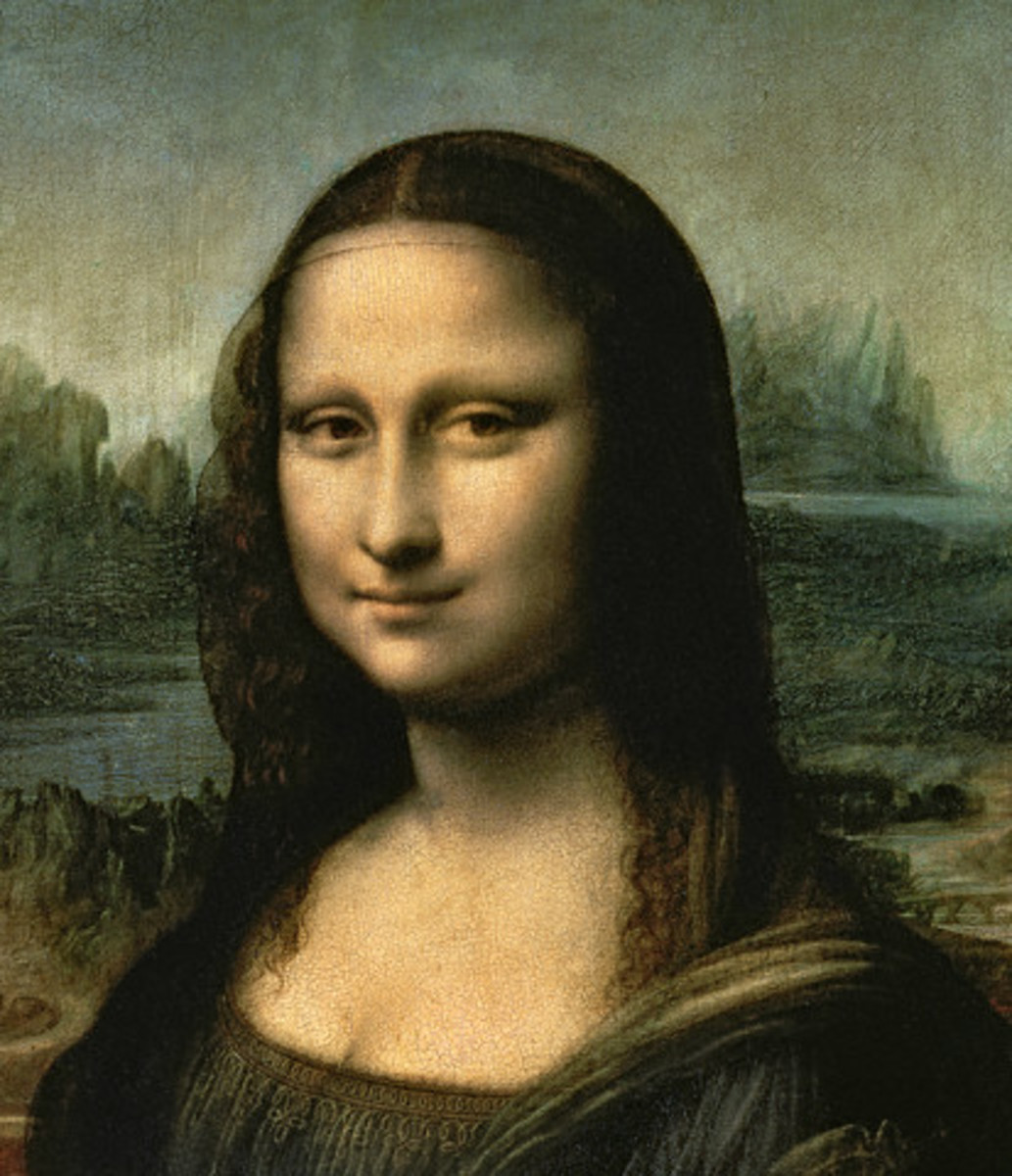Fig. 4. Is beauty hidden in the numbers? Detail of Mona Lisa.

## Fibonacci numbers and the golden ratio

It is said that two quantities are in the golden ratio when the ratio of the larger quantity to the smaller one equals the ratio between the sum of two quantities and the larger one. If the larger quantity is marked as a and the smaller one as b, than for the golden ratio φ it worth

(a+b)/a = a/b = φ

The solution of this equation is the irrational number φ=1.61803398..., and this ratio appears in many situation in nature, architecture and art in general.

Considering some remarkable paintings as Mona Lisa and The Last Supper from Leonardo da Vinci, one can notice that certain parts (in a rectangle shape) of the painting are just in the golden ratio (meaning longer side of the rectangle to another one, so we called it golden rectangle). Mona Lisa’s face actually appears in the golden rectangle and which is understood as the main reason of Mona Lisa’s extraordinary beauty. In case of the masterpiece The Last Supper the golden ratio can be noticed in several places.

Let now see ratio between two consecutive Fibonacci numbers, having in mind value of the golden ratio (1.61803398…):

2/1=1, 3/2=1.5, 5/3=1.666..., 8/5=1.6, 13/8=1.625, 21/13=1.61538...

Thus, the irrational number φ can be approximated by the fractions Fn+1/Fn, and we usually said that the ratio between two consecutive Fibonacci numbers is the golden ratio. Now it is clear that also Fibonacci rectangle is a golden rectangle.

## Cassini identity

The Figure 5 shows a geometric paradox. The rectangle of dimensions 13 x 5 and the square of dimension 8 x 8 are split into 4 parts as it is depicted. Both of the shapes consist of two triangles and two trapezoids. It seems that triangles as well as shapes are mutually equal which should means equal areas of the rectangle and the square. On the other hand, according to its dimension the area of rectangle should be 65 whereas the area of square should be 64. How to explain it? Where one small box (1 x 1) is lost?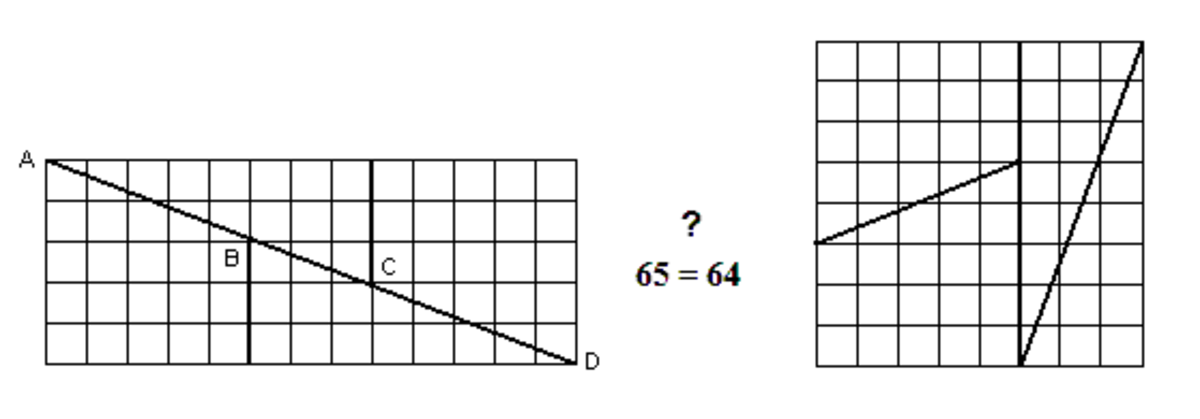Fig. 5. Illustration of Cassini identity. There are two triangles and two trapezoids in both shapes. Where one box of area 1 is missing?

Let note firstly that the length of sides of rectangular and square are the Fibonacci numbers 5, 8 and 13. Furthermore, it holds 13 x 5 -8 x 8 = 1. It can be proven that this worth for every three consecutive Fibonacci numbers. This property of Fibonacci numbers, expressed by the relation

Fn+1 x Fn-1 – Fn2 = (-1)n

is known as Cassini identity; named by French/Italian astronomer J.D.Cassini (1625-1712).

Thus, in order to set the task above any three consecutive Fibonacci numbers can serve as sides length. The difference between rectangular and square areas will always be 1.

The solution of the placed problem: the points A, B, C, D actually forms tiny parallelogram of the area 1.

The Cassini identity has a nice generalization. Namely, for and two Fibonacci numbers holds

Fn2 – Fn-r x Fn+r = (-1)n-r Fr2,

the rule known as Catalan's identity.

## Binet's Formula for the nth Fibonacci number

Let assume that one wants to know what is the 30th Fibonacci number. One possible approach to the solution is obvious: to start with F1=1 , F2=1,... and using the defining equation to calculate value of F30. However, there is a more elegant way for calculation of Fn, providing the result directly. This is known Binet's formula for the nth Fibonacci number. The formula is named after a French mathematician and astronomer J.P.M Binet (1786-1856).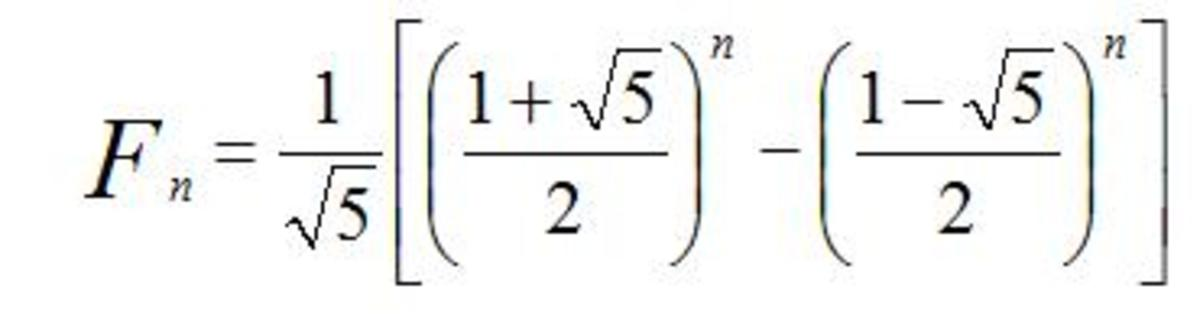## Check your knowledge and have fun! :-)

For each question, choose the best answer. The answer key is below.

1. What is the next number in sequence 3, 5, 8, 13,...?
• 14
• 19
• 21
2. Leonardo Fibonacci is also known as ... ?
• Leonardo of Pisa
• Leonardo of Bologna
• Leonardo of Roma
3. The ratio between two consecutive Fibonacci numbers tends to.. ?
• mathematical constant pi
• golden ratio
• the ratio depend on the chosen numbers
4. How many spirals a sunflower typically has?
• 21 in one direction and 35 in the other
• 34 one direction and 55 in the other
• 610 one direction and 987 in the other
5. Catalan identity reduces to Cassini when r equals ... ?
• n
• 1
• n-1

### Answer Key

1. 21
2. Leonardo of Pisa
3. golden ratio
4. 34 one direction and 55 in the other
5. 1

## Comments

flysky (author) from Zagreb, Croatia on July 18, 2015:

Yes, indeed! Especially it is the case with Fibonacci numbers, that are so present "everywhere". The deeper connection is the golder ratio (divine proportion) that is also hidden among Fibonacci numbers, i.e. the ratio between two consecutive Fibonacci numbers tend to – the golden ratio :-)

Many thanks for your comment !

Mel Carriere from Snowbound and down in Northern Colorado on July 18, 2015:

Very good lesson in mathematics, and how mathematics truly are the language of the universe.

flysky (author) from Zagreb, Croatia on January 01, 2012:

Thank you very much!

liftingmaniac on December 26, 2011:

A great read! Especially for someone like me who is interested in the properties of numbers.

flysky (author) from Zagreb, Croatia on July 31, 2011:

Thank you Habmath, very much! My experience with the students is the same; and this article is a short summary of materials I prepared for students.

HABMATH on July 11, 2011:

Great hub.I always try to introduce a few problems with Fibonacci numbers and the golden ratio in my Grade 11 math classes and the students are fascinated.

aayush on June 06, 2011:

tell me 10 examples of fibonacci

nextstopjupiter from here, there and everywhere on October 29, 2010:

The whole nature from the atom to the universe is based on Fibonacci fractals, to learn more watch this video http://www.youtube.com/watch?v=bE2EiI-UfsE

Thanks for this interesting hub!

flysky (author) from Zagreb, Croatia on September 06, 2010:

Hi PWalker! Here I primarly explain mathematical backgroung – since this is my field (togather with physics). Your experience is really interesting and impressive, and thank you for sharing it and enriching this topic. :-)

PWalker281 on September 05, 2010:

Very informative! I learned how to apply Fibonacci sequences to make color changes in crocheted fabric at a crochet conference some years ago. The resulting stripes are pleasing to the eye, even with colors that don't normally "match."

flysky (author) from Zagreb, Croatia on September 04, 2010:

Thank you, WestOcean. Not yet, but I heard of it. Would you recommend it?

WestOcean from Great Britain on September 03, 2010:

Great hub. Have you ever seen the movie "Pi"?